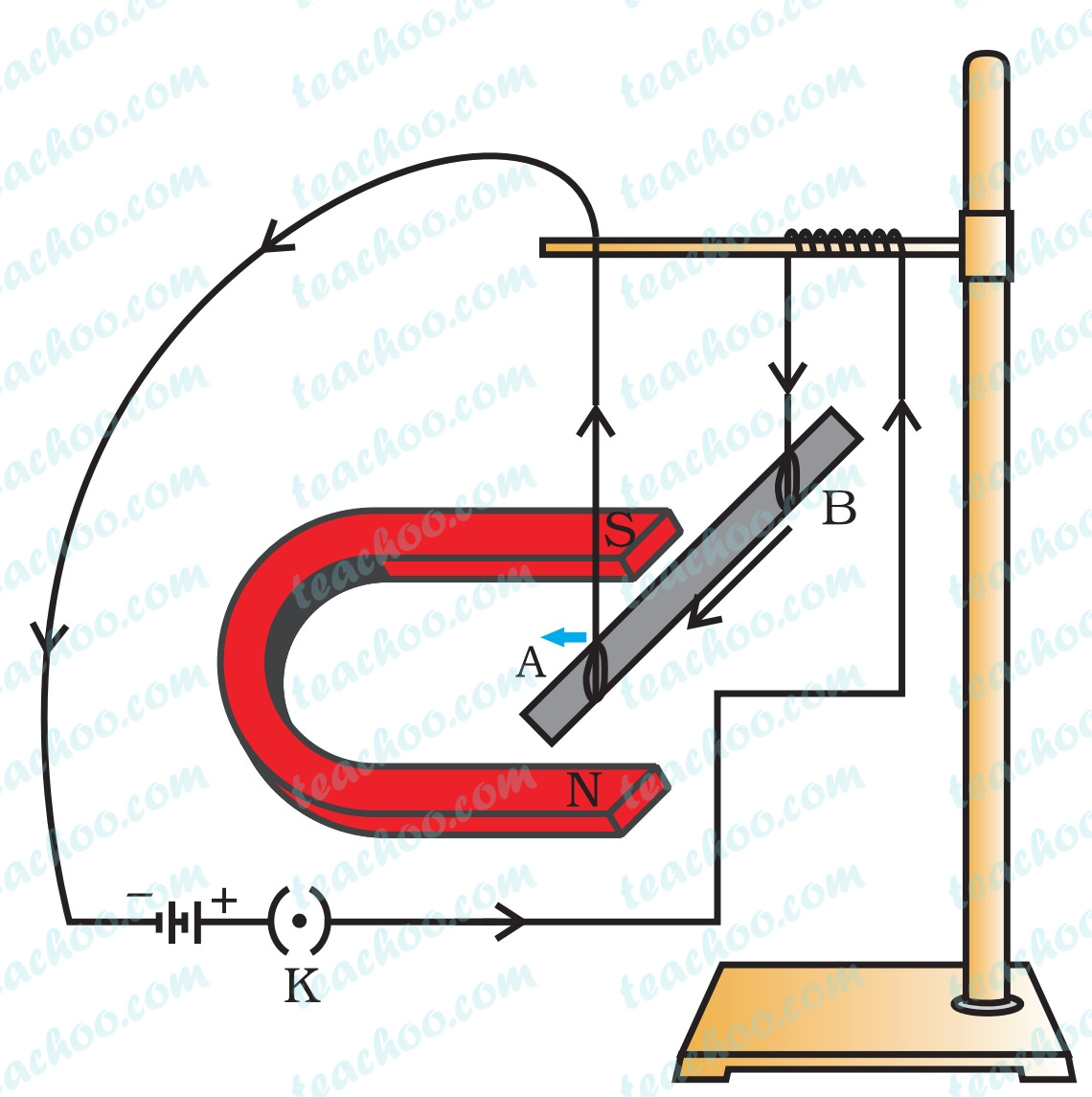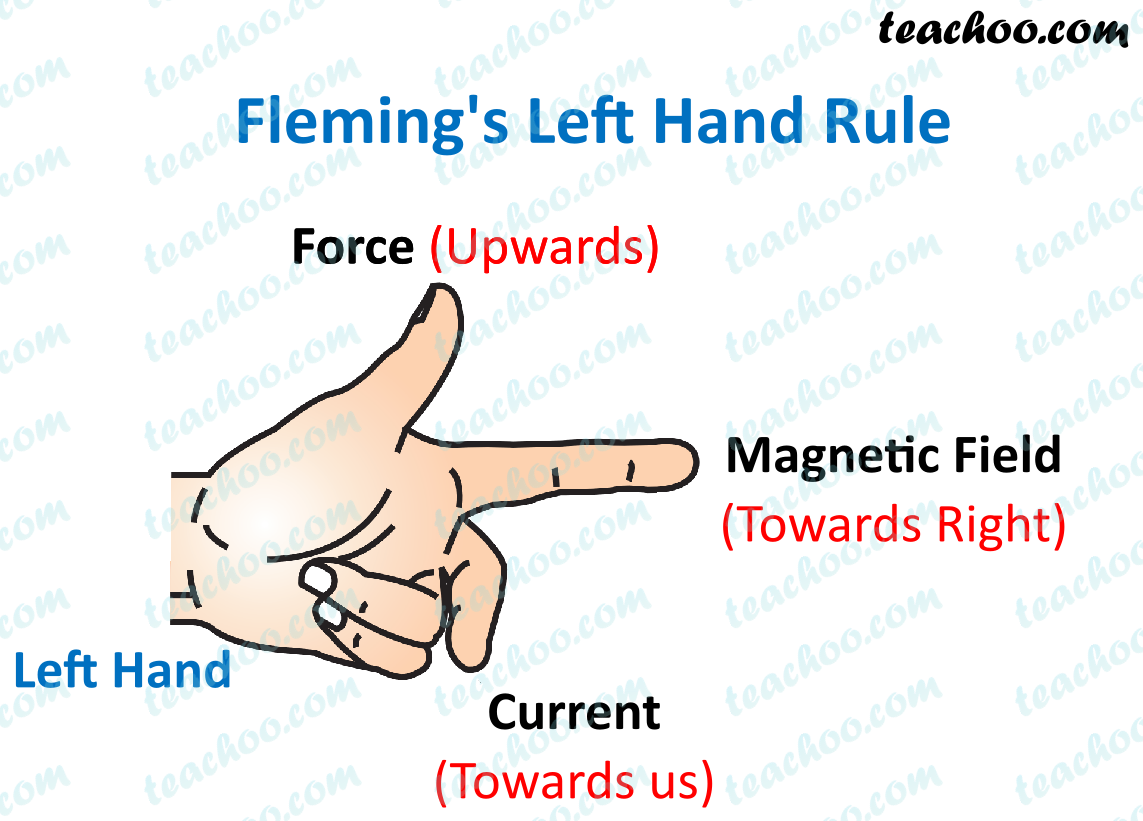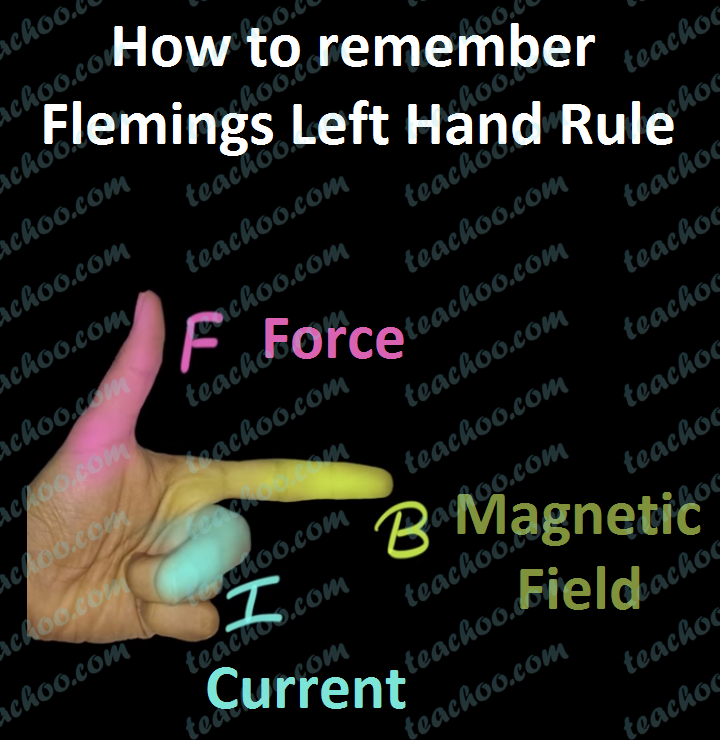Concepts

Class 10
Chapter 13 Class 10 - Magnetic Effects of Electric Current

We have learned that

Current Carrying Wire produces a magnetic field.

Now, this magnetic field exerts a force on the conductor.

So, current causes magnetic field which causes force due to magnetic field

## Activity to demonstrate Force due to Magnetic FieldLet's perform this experiment

• Take an aluminium rod
• Connect its 2 ends with wire
• Suspend it on a rod
• Take a strong horseshoe magnet
• Place it such that Aluminium rod is between north and south pole of the magnet
• Now, pass current through the wires after connecting it to a battery and a key.
• Rod is displaced towards the left
• If we reverse direction of current
• Rod is now displaced towards the right
• If we reverse direction of magnetic field (reverse poles of magnet)
• Rod is now displaced again towards left.

So, in the experiment we see that

• More current means more force
• Force is maximum when wire is perpendicular to magnetic field (i.e. when Current and Magnetic Field are at 90)
• Direction of Force depends on both - direction of current and direction of magnetic field

## Direction of Force depends on

From the experiment, we see that

Direction of Force acting on current carrying conductor (wire) depends upon

• Direction of Current - If we change direction of flow of current, direction of force on wire also changes
• Direction of Magnetic Field - If we change poles of magnet, direction of force on wire also changes

## When is force acting on Current Carrying Wire highest?

It is found by experiments that

Force Acting on Current Carrying Wire is highest when

Direction of Current is at 90 degrees (perpendicular) to Direction of Magnetic Field

Hence, the displacement of the conductor is largest when the magnetic field is perpendicular to the direction flow of current.

## How to Find Direction of Force Acting on Current Carrying Wire?

It is found with the help of Fleming Left Hand Rule

On our left hand,

We put our Thumb, Index finger and Middle Finger perpendicular to each other as shown belowThen,

• Index Finger represents Direction of Magnetic Field
• Middle Finger represents Direction of Current
• Our Thumb shows direction of Force

To remember this, remember FBI

• F - Force
• B - Magnetic Field
• I - CurrentSome solved Numericals on Fleming's Left Hand Rule are given in the Questions below

## Practical Uses of this Force

Force due to Magnetic field is used in construction and functioning of Electric Motors

These electric motors are used in various electric appliances like fans, refrigerators, mixtures etc

## Questions

NCERT Question 9 - When is the force experienced by a current–carrying conductor placed in a magnetic field largest?

NCERT Question 10 - Imagine that you are sitting in a chamber with your back to one wall. An electron beam, moving horizontally from back wall towards the front wall is deflected by a strong magnetic field to your right side. What is the direction of magnetic field?

NCERT Question 15 (ii) - State the rule to determine the direction of a

force experienced by a current-carrying straight conductor placed in a magnetic field which is perpendicular to it, and

Q1 Page 231 - Which of the following property of a proton can change while it moves freely in a magnetic field? (there may be more than one correct answer.)

(a) Mass            (b) Speed

(c) velocity         (d) momentum

Q1 Page 233 - State Fleming’s left-hand rule.

Example 13.2 - An electron enters a magnetic field at right angles to it, as shown in Fig. 13.14. The direction of force acting on the electron will be

1. to the right.
2. to the left.
3. out of the page.
4. into the page.

Learn in your speed, with individual attention - Teachoo Maths 1-on-1 Class